# Electronics and Communication Engineering - Analog Electronics

21.

An engineer designs an amplifier to have a voltage gain of 60, but when constructed it only had a gain of 50. Which of the following will increases from this type of operation?

 A. Stability B. Bandwidth C. Distortion D. Input resistance

Explanation:

No answer description available for this question. Let us discuss.

22.

For a transistor if adc = 0.98 and emitter current IE is 2 mA, then base current will be

 A. 10 μA B. 40 μA C. 80 μA D. 200 μA

Explanation:

No answer description available for this question. Let us discuss.

23.

Assertion (A): Linear ICs include audio amplifier, wideband amplifier, RF amplifier and IF amplifier

Reason (R): The error voltage in a feedback circuit is very small

 A. Both A and R are correct and R is correct explanation for A B. Both A and R are correct but R is not correct explanation for A C. A is correct R is wrong D. A is wrong R is correct

Explanation:

No answer description available for this question. Let us discuss.

24.

The given circuit has a feedback factor of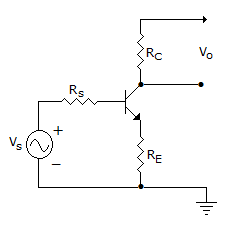A.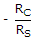B.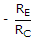C.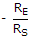D.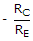Explanation:

No answer description available for this question. Let us discuss.

25.

Assertion (A): The horizontal intercept of dc load line is the same as ideal cut off point

Reason (R): When a transistor is in cut off region, collector current is zero

 A. Both A and R are correct and R is correct explanation for A B. Both A and R are correct but R is not correct explanation for A C. A is correct R is wrong D. A is wrong R is correct